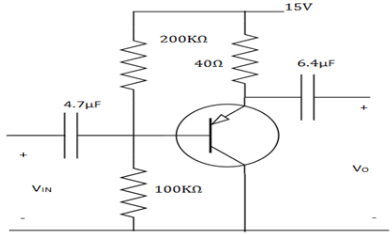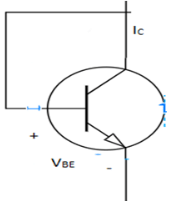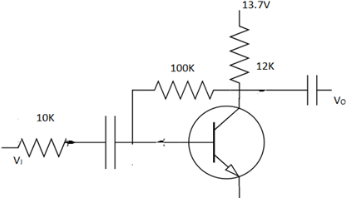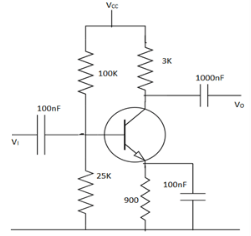# Test: Biasing Parameters

## 10 Questions MCQ Test Analog Electronics | Test: Biasing Parameters

Description
Attempt Test: Biasing Parameters | 10 questions in 10 minutes | Mock test for Electrical Engineering (EE) preparation | Free important questions MCQ to study Analog Electronics for Electrical Engineering (EE) Exam | Download free PDF with solutions
QUESTION: 1

### The current gain of BJT is_________

Solution:

We know, current gain AV=hfe. In π model, hfe is referred to β.
We know, ri= β/gm.
From this, β=rigm.

QUESTION: 2

### For the amplifier circuit of fig. The transistor has β of 800. The mid band voltage gain VO/VI of the circuit will be_________​Solution:

The circuit is PNP transistor, collector coupled amplifier. The voltage gain is unity for a CC amplifier. Hence on observation, the CC amplifier gives a unity gain.

QUESTION: 3

### In a bipolar transistor at room temperature, the emitter current is doubled the voltage across its base emitter junction_________

Solution:

The change in voltage with temperature can be found by, V(T) = 2.3m(∆T)VO . In a bipolar transistor at room temperature if the emitter current is doubled the voltage across its base emitter junction thereby doubles.

QUESTION: 4

A common emitter transistor amplifier has a collector current of 10mA, when its base current is 25µA at the room, temperature. What is input resistance?

Solution:

We know, β/gm=ri
= (IC/IB)/(IC/VT)=VT/IB=25m/25µ=1k.

QUESTION: 5

For an NPN transistor connected as shown in below, VBE=0.7V. Give that reverse saturation current of junction at room temperature is 10-13A, the emitter current is_________​Solution:

When the collector and base are shorted, the transistor behaves as a normal diode. So, the diode equations imply. IE=IO(eV/V0-1). We get, IE=49mA.

QUESTION: 6

The voltage gain of given circuit below is_________​Solution:

The gain for the given circuit can be found by, AV=RF/RS
=100K/10K=10.

QUESTION: 7

A small signal source V(t)=Acos20t+Bsin10000t, is applied to a transistor amplifier as shown. The transistor has β=150 and hie=3KΩ. What will be the VO?​Solution:

AV=-hfe RLI/hie=3*150/3=-150. So, VO=-150V(t)
But cos20t has low frequency so capacitors are open circuited. Only, the sine component is allowed.
So, Vo =-150Bsin10000t.

QUESTION: 8

Which of the following statements are correct for basic transistor configurations?

Solution:

The CE amplifier has moderate input and output impedances. The CC amplifier has unity voltage gain. The common ba se amplifier has a unity current gain and high voltage gain.

QUESTION: 9

The collector current is 2.945A and α=0.98. The leakage current is 2µA. What is the emitter current and base current?

Solution:

(IC – ICBO)/α=IE
= (2.945-0.002)/0.98=3mA.
IE=IC+IB . So, IB=3-2.495=0.055mA=55µA.

QUESTION: 10

The change in collector emitter voltage from 6V to 9V causes increase in collector current from 6mA to 6.3mA. Determine the dynamic output resistance.

Solution:

ro=∆VCE/∆IC
=3/0.3m=10kΩ.Use Code STAYHOME200 and get INR 200 additional OFF Use Coupon Code